# NCERT Solutions for Class 7 Maths Chapter 2 Fractions and Decimals

NCERT solutions for class 7 maths chapter 2 Fractions and Decimals- We have learnt fractions and decimals with their addition and subtraction in our earlier classes. In the solutions of NCERT class 7 chapter 2 fractions and decimals, we will learn questions related to multiplication and division of fractions and decimals. There are 7 exercises and topic wise questions in the chapter. All these questions are explained in the CBSE NCERT solutions for class 7 maths chapter 2 fractions and decimals. The study of fractions includes mixed fractions, proper fraction and improper fraction with their addition and subtraction, equivalent fractions, comparison of fractions, ordering of fractions and representation of fractions on a number line. Our study of decimals included their addition and subtraction, their comparison and the representation of decimals on a number line. NCERT solutions are the detailed explanation of each and every question of NCERT textbook and this helps to score good marks in the class exams. In NCERT solutions for class 7 maths chapter 2 fractions and decimals, we will study multiplication and division of decimals and fractions. Some questions related to real-life situations are also explained in the NCERT solutions for class 7 chapter 2 fractions and decimals. Here you will get solutions to all five exercises of this chapter.

Exercise:2.1

Exercise:2.2

Exercise:2.3

Exercise:2.4

Exercise:2.5

## Topics of NCERT class 7 maths chapter 2 fractions and decimals-

2.1  Introduction

2.2  How well have you learnt about Fractions?

2.3  Multiplication of Fractions

2.3.1 Multiplication of a Fraction by a Whole Number

2.3.2  Multiplication of a Fraction by a Fraction

2.4  Division of Fractions

2.4.1  Division of the Whole Number by a Fraction

2.4.2  Division of a Fraction by a Whole Number

2.4.3  Division of a Fraction by Another Fraction

2.5 How well have you learnt about Decimal Numbers

2.6  Multiplication of Decimal Numbers

2.6.1  Multiplication of Decimal Numbers by 10, 100 and 1000

2.7  Division of Decimal Numbers

2.7.1  Division by 10, 100 and 1000

2.7.2  Division of a Decimal Number by a Whole Number

2.7.3 Division of a Decimal Number by another Decimal Number

## NCERT solutions for class 7 maths chapter 2 fractions and decimals topic 2.3.1

1.(a)    Find:

If the product is an improper fraction express it as a mixed fraction.

Given,

The product is a proper fraction.

1.(b)   Find:

If the product is an improper fraction express it as a mixed fraction.

Given the product

This is an improper fraction, so convert it into a mixed fraction.

.

1.(c)   Find:

If the product is an improper fraction express it as a mixed fraction.

Given, the product

This is a proper fraction.

1.(d)     Find:

If the product is an improper fraction express it as a mixed fraction.

Given the product:

This is an improper fraction, so we convert this into a mixed fraction. that is

.1.(i)   Find:

1.(ii)  Find :

.

As we know, of means multiply so,

And

And

1.   Find:

As we know in the multiplication of a fraction, the numerator of one fraction is multiplied by the numerator of other fraction, and same for denominator, hence,

1. Find :

As we know in the multiplication of a fraction, the numerator of one fraction is multiplied by the numerator of other fraction, and same for denominator, hence,

## CBSE NCERT solutions for class 7 maths chapter 2 fractions and decimals topic 2.3.2

## 1. Find :

As we know, The division of a number by a / b is equivalent to multiplication of that number with b / a. So,

## NCERT solutions for class 7 chapter 2 fractions and decimals topic 2.4.2

2.  Find:

As we know, The division of a number by a / b is equivalent to multiplication of that number with b / a. So,

## CBSE NCERT solutions for class 7 maths chapter 2 fractions and decimals topic 2.4.3

3.  Find:

As we know, The division of a number by a / b is equivalent to multiplication of that number with b / a. So,

## Solutions of NCERT class 7 chapter 2 fractions and decimals topic 2.6

1. Find:

As we know, The multiplication of the decimal number is just like multiplication of normal number, we just have to put decimal before a digit such that the number of digits after decimal should remain same.

In other words,

The Number of digits after the decimal should be the same before and after the multiplication.

So.

The products in descending order are:

## NCERT solutions for class 7 chapter 2 fractions and decimals topic 2.6.1

1.  Find:

As we know, The multiplication of the decimal number is just like multiplication of normal number, we just have to put decimal before a digit such that the number of digits after decimal should remain same.

In other words,

The number of digits after the decimal should be the same before and after the multiplication.

So.

## CBSE NCERT solutions for class 7 chapter 2 fractions and decimals topic 2.7.1

1. Find:

(i) 235.4 ÷ 10      (ii) 235.4 ÷100      (iii) 235.4 ÷ 1000

As we know, while dividing a number by 10, 100 or 1000, the digits of the number and the quotient are same but the decimal point in the quotient shifts to the left by as many places as there are zeros over 1.

So,

(i) 235.4 ÷ 10    =  23.54

(ii) 235.4 ÷100     = 2.354

(iii) 235.4 ÷ 1000  = 0.2354

## NCERT solutions for class 7 chapter 2 fractions and decimals topic 2.7.2

As we know, dividing by a number is equivalent to multiplying by the reciprocal of that number. So

(i) 35.7 ÷ 3

(ii) 25.5 ÷ 3

As we know, dividing by a number is equivalent to multiplying by the reciprocal of that number. So

(i) 43.15 ÷ 5

(ii) 82.44 ÷ 6

4.    Find:

(i) 15.5 ÷ 5

(ii) 126.35 ÷ 7

As we know, dividing by a number is equivalent to multiplying by the reciprocal of that number. So

(i) 15.5 ÷ 5

(ii) 126.35 ÷ 7

## Solutions of NCERT class 7 chapter 2 fractions and decimals topic 2.7.3

1. Find:

As we know, in the dividing of decimal, we first express the decimal in term of fraction and then divides it, So

## NCERT solutions for class 7 maths chapter 2 fractions and decimals exercise 2.1

1.  Solve:

(i)                          (ii)                    (iii)                    (iv)

(v)            (vi)            (vii)

As we know we have to make the denominator the same in order to add or subtract the fractions. So,

(i)

(ii)

(iii)

(iv)

(v)

(vi)

(vii)

(i)                (ii)

(i)

As 14 < 24 < 42

(ii)

As 14 < 30 < 49

Given,

Length of the rectangle :

Width of the rectangle :

Now, As we know,

Perimeter of the rectangle = 2 x ( length + width )

So,

The perimeter of the given rectangle :

Now let's make the denominator of both fractions equal.

Hence, the perimeter of the rectangle is .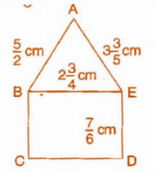The perimeter of  = AB + BE + AE

Noe, The LCM of 2,4 and 5 is 20. So let's make the denominator of all fraction, 20.

So,

The perimeter of  :

Now,

The perimeter of rectangle BCDE = 2 x ( BE + ED )

The LCM of 4 and 6 is 12. So let's make the denominator of both fractions equal to 12.

Hence The perimeter of the Triangle is  and the perimeter of Rectangle is  .

Now, we have

LCM of 20 and 6 is 60, so let's make denominator of both fraction equal to 60.

So,

And

Now, Since 531 > 470

Area of Triangle > Area of Rectangle.

Given, the width of the picture =   cm.

The maximum width of the picture which can fit in the frame =  cm.

Hence the length Salil should trim :

Now LCM of 5 and 10 is 10. So, let's make the denominator of both fractions equal to 10. So,

Hence Salil should cut 3/10 cm of the picture in order to fit it in the frame.

Given,

Part of Apple eaten by Ritu =      .

Part of Apple eaten by Somu:

LCM of 1 and 5 is 5. So making the denominator of both fraction equal to 5, we get

Hence, The Part of Apple eaten by Somu is 2/5.

Now, As

Hence, Ritu had a larger share of the apple by  part.

Time taken by Michal in coloring =    hours.

Time taken by Vaibhav in coloring =   hours.

The LCM of 12 and 4 is 12. So making the denominator of both fraction equal to 12, we get,

As

For calculating by how much, we do Subtraction

Vaibhav worked longer by the fraction .

## CBSE NCERT solutions for class 7 maths chapter 2 fractions and decimals exercise 2.2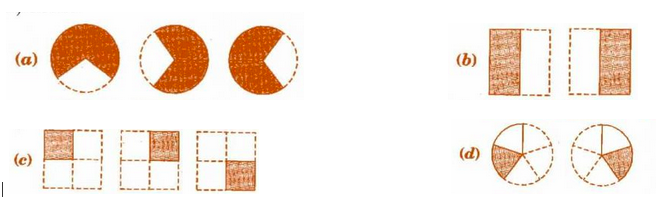i)

(d) represents two circles with 1 part shaded out of 5 part So, it represents

.

ii) b represents two square one part out of two of both square are shaded, So it represents

(iii)

(a) represents 3 circles 2 parts out of three of all circles are shaded. So it represents

(iv)

(c) represents 3 square with one part out of four-part shaded in each square hence it represents

.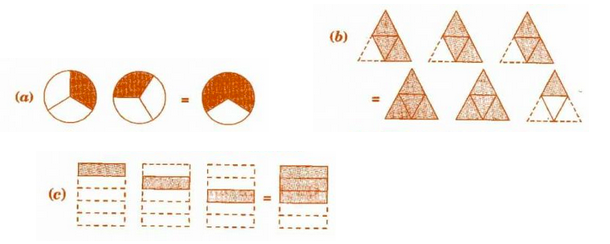As in option (c), In the Left-hand side, there are three figures in which the one part out of three-part is shaded and in the right-hand side, three out of five portions are shaded.

Hence this represents

.

Option (a) represents the equation pictorially.

Option (b) represents this equation pictorially.

On Multiplying, we get

On Multiplying, we get

On Multiplying, we get

On Multiplying, we get

On Multiplying, we get

On Multiplying, we get

On Multiplying, we get

Converting this into a mixed fraction, we get

On Multiplying, we get

On multiplying, we get

Converting this into a mixed fraction,

.

On multiplying, we get,

.

of the circles in box (a)                   of the triangles in box (b)

of the squares in box (c).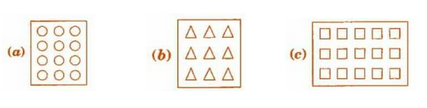1) In figure a there are 12 circles: half of 12 = 6

2) In figure b there are 9 triangles: 2/3 of 9 = 6

3) In figure c there are 15 triangles: 3/5 of 15 = 95.   Find:

On Multiplying we get,

On multiplying, we get

On Multiplying, we get

On multiplying, we get

On multiplying, we get

On multiplying, we get

On Multiplying, we get,

On Multiplying, we get

Converting This into Mixed Fraction,

On Multiplying, we get

Converting This into Mixed Fraction,

On multiplying, we get

Converting it into a mixed fraction, we get

On multiplying, we get

Converting it into a mixed fraction,

Multiplying them, we get

Now, converting the result fraction we got to mixed fraction,

On multiplying, we get

Converting this into a mixed fraction, we get

7.   Find:

As we know that of is equivalent to multiply,

As we know that of is equivalent to multiply,

As we know that of is equivalent to multiplication, so

As we know that of is equivalent to multiplication, so

(i) How much water did Vidya drink?
(ii) What fraction of the total quantity of water did Pratap drink?

Given

Total water  = 5 litre.

i) The amount of water vidya consumed :

Hence vidya consumed 2 liters of water from the bottle.

ii) The amount of water Pratap consumed :

Hence, Pratap consumed 3 liters of water from the bottle.

## 1.  Find:

As we know, the term "of " means multiplication. So,

As we Know, in the multiplication of fraction, the numerator gets multiplied with numerator and denominator gets multiplied by the denominator. So,

.

As we know in the multiplication of fractions, the numerator is multiplied with numerator and denominator is multiplied by the denominator.

So,

Now, As we Know, When the numerator of two fractions is the same the fraction with lesser denominator is the bigger fraction. So,

Thus,

.

As we know, When the denominator of two fractions are the same, the fraction with the bigger numerator is the bigger fraction, so,

Thus,

.

distance between two adjacent saplings =

distance between the first and the last sapling :

.

Number of time spent in one day =   hour

The number of time spent in 6 days :

Hence  are required by Lipika to complete the book.

Distance covered in 1 liter petrol  = 16 km

The distance that will be covered in  liter petrol.

Hence 44 km can be covered using   liter petrol.

(ii) The simplest form of the number obtained in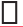is _________.

(b)  (i) Provide the number in the box, such that= .

(ii) The simplest form of the number obtained inis _________.

As we Know, That in the multiplication of fraction, the numerator of both fraction are multiplied to give numerator of new fraction, and denominator of both fractions is multiplied to give the denominator of answer fraction. So,= .

We have to multiply 5 with 2 in the numerator to get numerator equal to 10 and 10 with 3 in the denominator to get 30. So,

The Simplest Form :

## NCERT solutions for class 7 maths chapter 2 fractions and decimals exercise 2.4

1.  Find:

As we know, The division of a number by a / b is equivalent to multiplication of that number with b / a. So,

As we know, in the reciprocal of any fraction, the numerator and denominator get exchanged. Basically, we flip the number upside down. So

As numerator is greater than the denominator, it is an improper fraction.

As numerator is greater than the denominator, it is an improper fraction.

As the Denominator is greater than Numerator, it is a proper fraction.

As the Denominator is greater than Numerator, it is a proper fraction.

As the Denominator is greater than Numerator, it is a proper fraction.

It is an integer and hence a  whole Number.

It is an integer and hence a  whole Number.

3. Find:

As we know, The division of a number by a / b is equivalent to multiplication of that number with b / a which is also called the reciprocal of a / b. So,

4.  Find:

As we know, The division of a number by a / b is equivalent to multiplication of that number with b / a which is also called the reciprocal of a / b. So,

## 1.  Which is greater?

(i) 0.5 or 0.05       (ii) 0.7 or 0.5       (iii) 7 or 0.7
(iv) 1.37 or 1.49    (v) 2.03 or 2.30    (vi) 0.8 or 0.88.

As we know, Any decimal is equivalent to the number without decimal divided by 10 x (number of integers after the decimal). in other words,

So,

(i) 0.5 >  0.05

(ii) 0.7 > 0.5

(iii) 7 > 0.7

(iv) 1.37 < 1.49

(v) 2.03 < 2.30

(vi) 0.8 < 0.88.

(ii) Express 35 mm in cm, m and km

As we know,

1 centimeter = 10 millimeter

1 meter = 100 centimeter.

And

1 kilometer = 1000 meter

So,

i) 5 cm

ii) 35 mm

(i) 200 g      (ii) 3470 g     (iii) 4 kg 8 g

As we know,

1 kg = 1000 g.

So,

i) 200 g

(ii) 3470 g

(iii) 4 kg 8 g

Decimal in their expanded form are

(i) 20.03

(ii) 2.03

(iii) 200.03

(iv) 2.034

(i) 2.56

2 is in one's position.

(ii) 21.37

2 is in ten's position

(iii) 10.25

2 is in one-tenths position

(iv) 9.42

2 is in one-hundredths position.

(v) 63.352.

2 is in one-thousandth's position.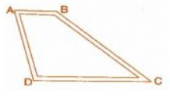Total distance travelled by Dinesh = AB + BC

=  7.5km + 12.7km

= 20.2 km

Total distance travelled by Ayub = AD + DC

= 9.3km + 11.8 km

=  21.1 km

Hence Ayub travelled More distance than Ayub as 21.1 > 20.2

The difference between path travelled by them = 21.1km - 20.2 km

= 0.9 km.

Hence Ayub travelled 0.9 km more than Dinesh.

For comparing two quantities, we should make their unit the same first. So,

Fruits bought by Shyama in kg =  5 kg + 300 g + 3 kg + 250 g

=  8 kg + 550 g

=  8 kg + 0.55 kg

= 8.55 kg.

Fruits bought by Sarala in kg  = 4 kg + 800 g + 4 kg + 150 g

= 8 kg + 950 kg

= 8 kg + 0.95 kg

= 8.95 kg.

Hence Sarala bought more fruits as 8.95 > 8.55

The difference between the amount of Fruits they bought =  8.95 kg - 8.55 kg

= 0.4 kg

Difference = 42.6 km - 28 km

= 14.6 km

Hence 28  is 14.6 km less than 42.6.

## NCERT solutions for class 7 chapter 2 fractions and decimals exercise 2.6

1.  Find:

(i) 0.2 × 6          (ii) 8 × 4.6         (iii) 2.71 × 5       (iv) 20.1 × 4

(v) 0.05 × 7        (vi) 211.02 × 4   (vii) 2 × 0.86

As we know, The multiplication of the decimal number is just like multiplication of normal number, we just have to put decimal before a digit such that the number of digits after decimal should remain same.

In other words,

The number of digits after the decimal should be the same before and after the multiplication.

So.

(i) 0.2 × 6  = 1.2

(ii) 8 × 4.6  = 36.8

(iii) 2.71 × 5 = 13.55

(iv) 20.1 × 4 = 80.4

(v) 0.05 × 7  = 0.35

(vi) 211.02 × 4  = 844.08

(vii) 2 × 0.86 = 1.72

Given, Length of rectangle = 5.7 cm.

Width of rectangle =  3 cm

Area of the rectangle = Length x width

= 5.7 x 3

=  17.1 .

Hence Area of the rectangle is 17.1 .

3.  Find:

(i) 1.3 × 10      (ii) 36.8 × 10      (iii) 153.7 × 10      (iv) 168.07 × 10

(v) 31.1 × 100  (vi) 156.1 × 100  (vii) 3.62 × 100     (viii) 43.07 × 100

(ix) 0.5 × 10    (x) 0.08 × 10      (xi) 0.9 × 100       (xii) 0.03 × 1000

As we know, The multiplication of the decimal number is just like multiplication of normal number, we just have to put decimal before a digit such that the number of digits after decimal should remain same.

In other words,

The number of digits after the decimal should be the same before and after the multiplication.

So.

(i) 1.3 × 10  = 13

(ii) 36.8 × 10 = 368

(iii) 153.7 × 10  = 1537

(iv) 168.07 × 10 = 1680.7

(v) 31.1 × 100 = 3110

(vi) 156.1 × 100 =15610

(vii) 3.62 × 100 = 362

(viii) 43.07 × 100 = 4307

(ix) 0.5 × 10  = 5

(x) 0.08 × 10  = 0.8

(xi) 0.9 × 100  =  90

(xii) 0.03 × 1000 =30

Distance covered by two-wheeler in 1 liter of petrol = 55.3 km.

Distance two-wheeler will cover in 10 liters of petrol = 10 x 55.3 km

= 553 km

Hence two-wheeler will cover a distance of 553 km in 10 liters of petrol.

5. Find:

(i) 2.5 × 0.3      (ii) 0.1 × 51.7      (iii) 0.2 × 316.8      (iv) 1.3 × 3.1     (v) 0.5 × 0.05    (vi) 11.2 × 0.15    (vii) 1.07 × 0.02

(viii) 10.05 × 1.05       (ix) 101.01 × 0.01     (x) 100.01 × 1.1

As we know, The multiplication of the decimal number is just like multiplication of normal number, we just have to put decimal before a digit such that the number of digits after decimal should remain same.

In other words,

The number of digits after the decimal should be the same before and after the multiplication.

So.

(i) 2.5 × 0.3   = 0.75

(ii) 0.1 × 51.7  = 5.17

(iii) 0.2 × 316.8  =  63.36

(iv) 1.3 × 3.1   =   4.03

(v) 0.5 × 0.05  = 0.025

(vi) 11.2 × 0.15  = 1.680

(vii) 1.07 × 0.02  = 0.0214

(viii) 10.05 × 1.05  = 10.5525

(ix) 101.01 × 0.01  = 1.0101

(x) 100.01 × 1.1  = 110.11

NCERT solutions for class 7 chapter 2 fractions and decimals 2.7

1.   Find:

As we know, while dividing decimal, we first express the decimal in term of fraction and then divides it,

And

Dividing by a number is equivalent to multiplying by the reciprocal of that number. So

So

2.  Find:

As we know, When we divide a decimal number by 10, the decimal point gets shifted by one digit in the left.

So

3.  Find:

As we know while dividing a decimal number by 100, the decimal point gets shifted to left by two digits.

So

4.    Find:

As we know, while dividing a decimal number by 1000 we shift the decimal to left by 3 digits, So

5.   Find:

As we know, while dividing decimal, we first express the decimal in term of fraction and then divides it,

And

Dividing by a number is equivalent to multiplying by the reciprocal of that number.

So

Distance travelled by vehicle in 2.4 litres of petrol = 43.2 km

Distance travelled by vehicle in 1 litre of petrol:

Hence Distance travelled by vehicle in 1 litre is 18 km.

## NCERT Solutions for Class 7 Maths - Chapter-wise

 Chapter No. Chapter Name Chapter 1 Solutions of NCERT for class 7 maths chapter 1 Integers Chapter 2 CBSE NCERT solutions for class 7 maths chapter 2 Fractions and Decimals Chapter 3 NCERT solutions for class 7 maths chapter 3 Data Handling Chapter 4 Solutions of NCERT for class 7 maths chapter 4 Simple Equations Chapter 5 CBSE NCERT solutions for class 7 maths chapter 5 Lines and Angles Chapter 6 NCERT solutions for class 7 maths chapter 6 The Triangle and its Properties Chapter 7 Solutions of NCERT for class 7 maths chapter 7 Congruence of Triangles Chapter 8 NCERT solutions for class 7 maths chapter 8 comparing quantities Chapter 9 CBSE NCERT solutions for class 7 maths chapter 9 Rational Numbers Chapter 10 NCERT solutions for class 7 maths chapter 10 Practical Geometry Chapter 11 Solutions of NCERT for class 7 maths chapter 11 Perimeter and Area Chapter 12 CBSE NCERT solutions for class 7 maths chapter 12 Algebraic Expressions Chapter 13 NCERT solutions for class 7 maths chapter 13 Exponents and Powers Chapter 14 Solutions of NCERT for class 7 maths chapter 14 Symmetry

## Importance of NCERT solutions for class 7 chapter 2 fractions and decimals

• Now homework of the chapter becomes an easy breezy thing with CBSE NCERT solutions for class 7 chapter 2 fractions and decimals in hand.
• Solutions of NCERT class 7 chapter 2 fractions and decimals in hand helps students in self-evaluation
• The NCERT solutions for class 7 maths chapter 2 fractions and decimals are helpful in preparation for the exam. A similar type of questions can be expected for the class exams.